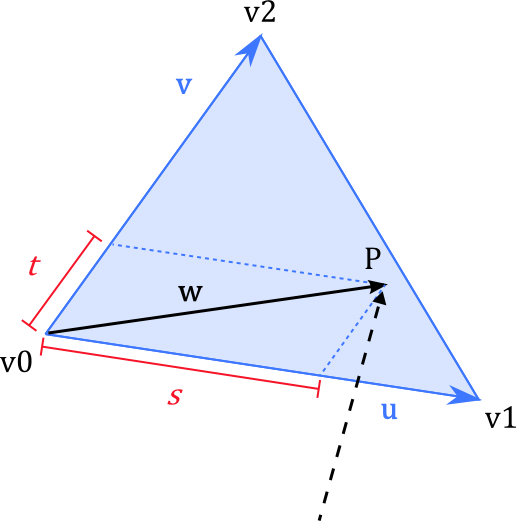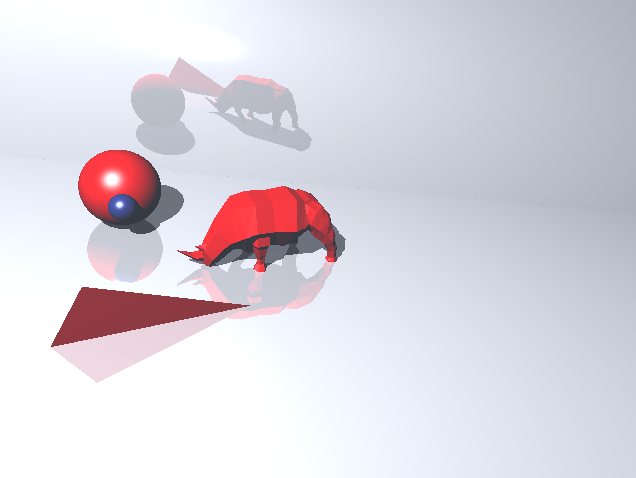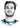# Howto Raytracer: Ray / Triangle Intersection Theory

Categories:

Besides sphere and plane intersections another important one is the ray / triangle intersection, because most 3D models consist of triangles or can be converted to such a representation. So let’s learn how to do it to be able to model some complex models.

A triangle $$T$$ can be represented by three points $$v0, v1, v2$$ that define a plane. So first, we check if the ray intersects this plane. I already did a tutorial on ray / plane intersection so I won’t cover it again. If there is such an intersection it means we just have to check if this hitpoint $$P$$ lies within the bounds of the triangle. For this we calculate a different representation of $$P$$ with respect to the triangle: As the point is on the plane, it can be written as $$P = v0 + su + tv$$ for some $$s,t$$ where $$u$$ and $$v$$ are the “edge vectors” incident to $$v0$$.Once we have found the values for $$s,t$$ the following has to be true for the point to be inside the triangle:

1. $$0 \leq s,t \leq 1$$
2. $$s + t \leq 1$$

These constraints on $$s,t$$ essentially define the triangle structure and all in all, the set of points of the triangle is \begin{aligned} T = {P \mid & P \in T_{\text{Plane}} \land P = v0 + s(v1-v0) + t(v2-v0) \land \\ & 0 \leq s,t, \leq 1 \land s + t \leq 1} \end{aligned}

Assume we have checked that $$P$$ lies inside the triangle’s plane, then we just have to solve $$P = v0 + su + tv$$ for $$s,t$$, or equivalent $$w = P - v0 = su + tv$$.

### Solving the equation

Unfortunately, $$w = su + tv$$ has two unknowns ($$s,t$$) in only one equation, so we have to use a little trick to get two equations out of this. The normal $$n$$ of the triangle can be computed by the cross product of $$u$$ and $$v$$. Let’s now consider the vector $$u^\perp$$ that is both perpendicular to $$u$$ and to $$n$$. This vector lies in the plane and can again be computed by using the cross product, i.e., $$u^\perp = n \times u$$. We can now (dot product-) multiply our equation $$w = su + tv$$ on both sides by $$u^\perp$$:

\begin{aligned} w = su + tv \\ w \cdot u^\perp = s u \cdot u^\perp + t v \cdot u^\perp \\ w \cdot u^\perp = t v \cdot u^\perp \end{aligned}

The term $$u \cdot u^\perp$$ is $$0$$, because by definition of $$u^\perp$$ it is perpendicular to $$u$$, and with it the $$s$$ vanishes and we have reduced the equation to only one unknown, so we solve it for $$t$$ by:

\begin{aligned} t = \frac{w \cdot u^\perp}{v \cdot u^\perp} \end{aligned}

We do a similar thing to compute $$s$$ by multiplying with $$v^\perp = n \times v$$: \begin{aligned} w = su + tv \\ w \cdot v^\perp = s u \cdot v^\perp + t v \cdot v^\perp \\ w \cdot v^\perp = s u \cdot v^\perp \\ s = \frac{w \cdot v^\perp}{u \cdot v^\perp} \end{aligned}

### Some optimizations

We can reduce the number of computations by seeing that the denominator used to compute $$s$$ is just the negative of the denominator for $$t$$:$$u\cdot v^\perp = u \cdot (n \times v) = v \cdot (u \times n) = v \cdot (-n \times u) = - v \cdot (n \times u) = - v \cdot u^\perp$$ This gives us the following equations:

\begin{aligned} s = \frac{w \cdot v^\perp}{u \cdot v^\perp} && v^\perp = n \times v && w = P - v0 \\ t = \frac{w \cdot u^\perp}{-u \cdot v^\perp} && u^\perp = n \times u & \end{aligned}

In the case of raytracing where we shoot a ray through the scene for every pixel, we can save a lot of computation time by caching some of these values. In fact, the only term that depends on the ray is $$w$$, whereas $$u^\perp, v^\perp, u \cdot v^\perp$$ only have to be computed once for each triangle.

Here’s some C# code that implements the ray/triangle intersection test:

using UnityEngine;

public class RTTriangle : RTObject
{
protected Vector3 v0, v1, v2;
protected Vector3 normal;
protected Vector3 u, v;
protected Vector3 uPerp, vPerp;
protected float denominatorST;

public RTTriangle(Vector3 v0, Vector3 v1, Vector3 v2, bool clockwise = false)
{
Init(v0, v1, v2, clockwise);
}

protected void Init(Vector3 v0, Vector3 v1, Vector3 v2, bool clockwise = false)
{
this.v0 = v0;
this.v1 = v1;
this.v2 = v2;
u = v1 - v0;
v = v2 - v0;

// Unity uses clockwise winding order to determine front-facing triangles
// Unity uses a left-handed coordinate system
// the normal will face the front
// if the direction of the normal is not important to you
// just remove the clockwise branching
normal = (clockwise ? 1 : -1) * Vector3.Cross(u, v).normalized;

uPerp = Vector3.Cross(normal, u);
vPerp = Vector3.Cross(normal, v);
denominatorST = Vector3.Dot(u, vPerp);
if (Mathf.Abs(denominatorST) < Mathf.Epsilon)
{
Debug.LogError("Triangle is broken");
return;
}
}

public override RayTracer.HitInfo Intersect(Ray ray)
{
RayTracer.HitInfo info = new RayTracer.HitInfo();

Vector3 d = ray.direction;
float denominator = Vector3.Dot(d, normal);

if (Mathf.Abs(denominator) < Mathf.Epsilon) return info;      // direction and plane parallel, no intersection

float tHit = Vector3.Dot(v0 - ray.origin, normal) / denominator;
if (tHit < 0) return info;    // plane behind ray's origin

// we have a hit point with the triangle's plane
Vector3 w = ray.GetPoint(tHit) - v0;

float s = Vector3.Dot(w, vPerp) / denominatorST;
if (s < 0 || s > 1) return info;    // won't be inside triangle

float t = Vector3.Dot(w, uPerp) / -denominatorST;
if (t >= 0 && (s + t) <= 1)
{
info.time = tHit;
info.hitPoint = ray.GetPoint(tHit);
info.normal = normal;
}

return info;
}
}


You can now import a 3D model consisting of triangles in .obj format, parse the triangles and do your ray test. This is what the result might look like, a lot better than just planes and spheres.# Hi, I'm Christoph Michel 👋I'm a , , and .

I mostly do and help small to mid-sized teams from all over the world 🌎 on both short and long-term projects on an independent contractor basis.

I strive for efficiency ⏱️ and therefore track many aspects of my life.